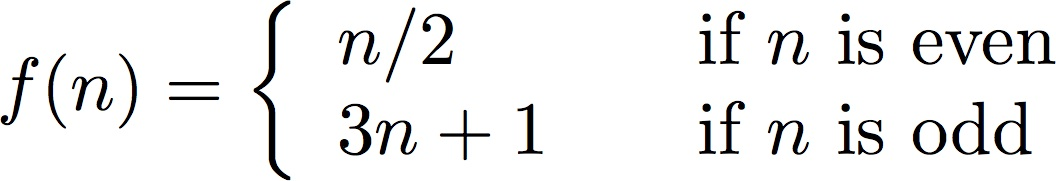# Extra Credit #2

Due: Thursday, February 8, 2018 at 11:59 p.m.
Points: 30

1. (30 points) Define the function:The Collatz conjecture says that, if you iterate this sequence for any initial value of n, then eventually the sequence will reach the number 1.

For a given number n, let k be the least number of iterations needed to reach the number 1 (excluding the initial value). Then k is called the total stopping time of n.

For example, if n = 29, then the sequence is:

29 88 44 22 11 34 17 52 26 13 40 20 10 5 16 8 4 2 1
and so the total stopping time of 29 is 18.

Write a program that takes as input a positive integer and prints both the sequence and the total stopping time for that integer. The output should look like:

29 88 44 22 11 34 17 52 26 13 40 20 10 5 16 8 4 2 1
The total stopping time for 29 is 18Matt Bishop Department of Computer Science University of California at Davis Davis, CA 95616-8562 USA